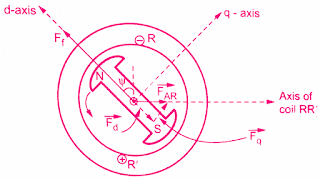# Two Reaction Theory – Salient Pole Synchronous Machine

0
412

### Two Reaction Theory

#### The component which is acting along the direct axis can be magnetizing or demagnetizing. The component which is acting along quadrature axis is crossly magnetizing.These components produce the effects of different kinds.The below figure shows the stator MMF wave and the flux distribution in the air gap along the direct axis and quadrature axis of the pole.The reluctance offered to the MMF wave is lowest when it is aligned with the field pole axis.This axis is called the direct axis of pole i.e. d-axis.The reluctance offered is highest when the MMF wave is oriented at 90° to the field pole axis which is called quadrature axis i.e. q-axis.

#### The phasor diagram of MMF wave positions in salient pole machine is shown below.#### It can be noted that the reactance offered to flux along the direct axis is less than the reactance offered to flux along quadrature axis.Due to this, the flux ΦAR is no longer along FAR or Ia.Depending upon the reluctances offered along the direct and quadrature axis, the flux ΦARlags behind armature current Ia.### Blondel two reaction theory phasor diagram Analysis :

#### From the Blondel two reaction theory phasor diagram shown in the below figure, the angles Ψ and 𝛿 values are unknown, though Vt, Ia, and  Φ values are known.Hence the location of Ef is also unknown.The components of Ia, Id, and Iq cannot be determined which are required to sketch the phasor diagram.Let us find out some geometrical relationships between the various quantities which are involved in the phasor diagram.#### The triangle ABC is the right-angled triangle.#### The point C can be located.Hence the direction of Ef is also known.Now triangle ODC is also right angle triangle,#### Hence the magnitude of Ef can be obtained by using the above equation

READ HERE  What is a Synchronous Machine? Synchronous Speed